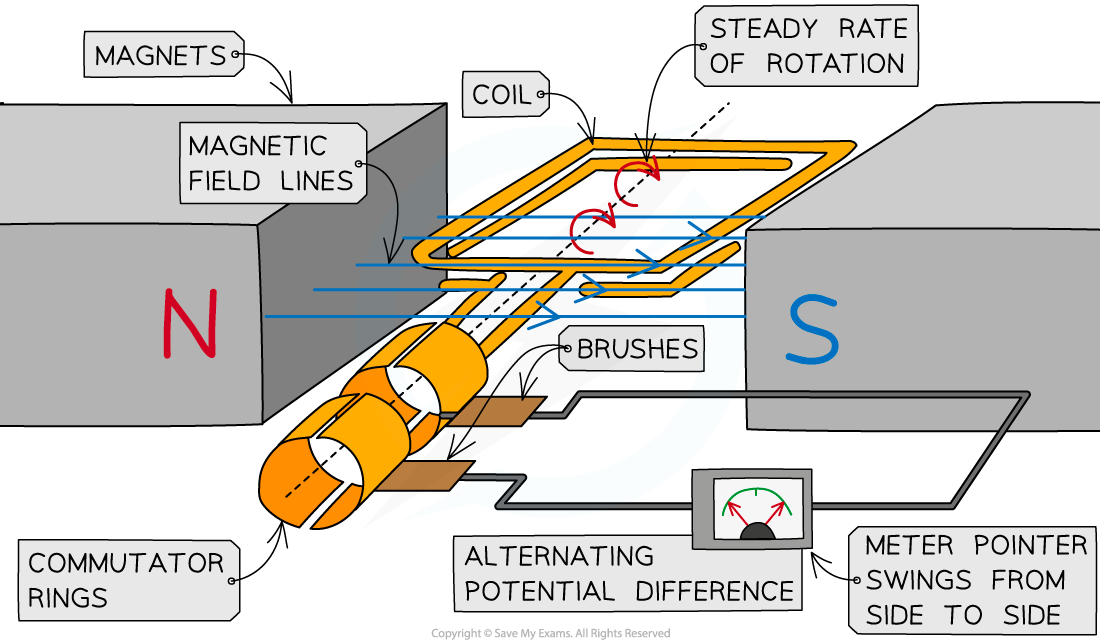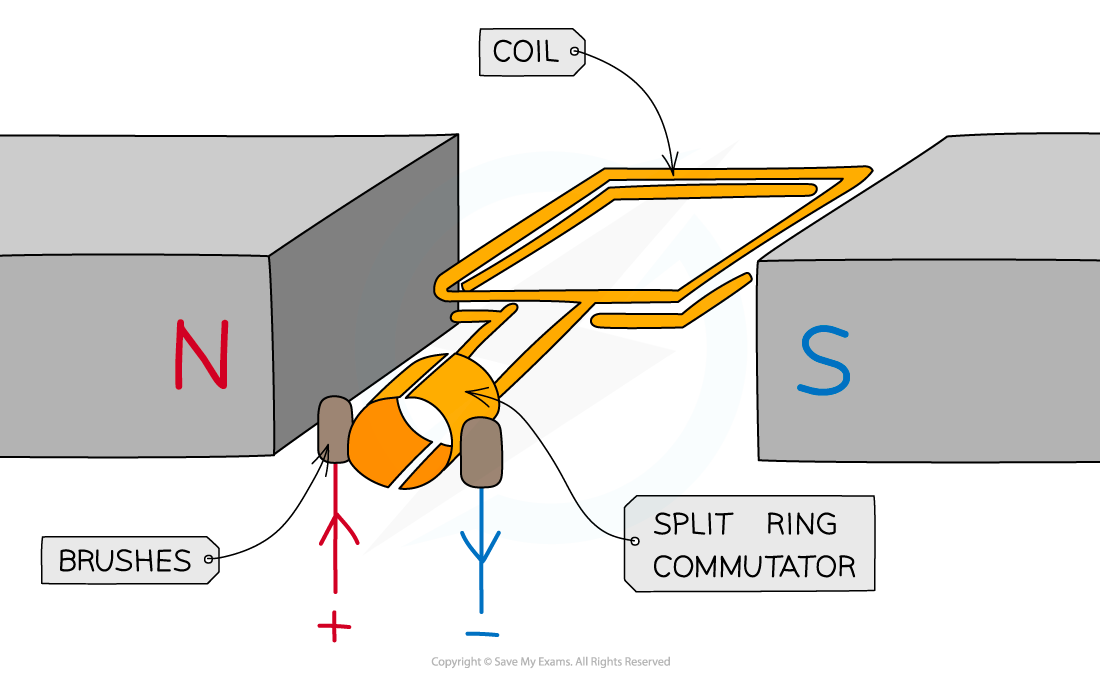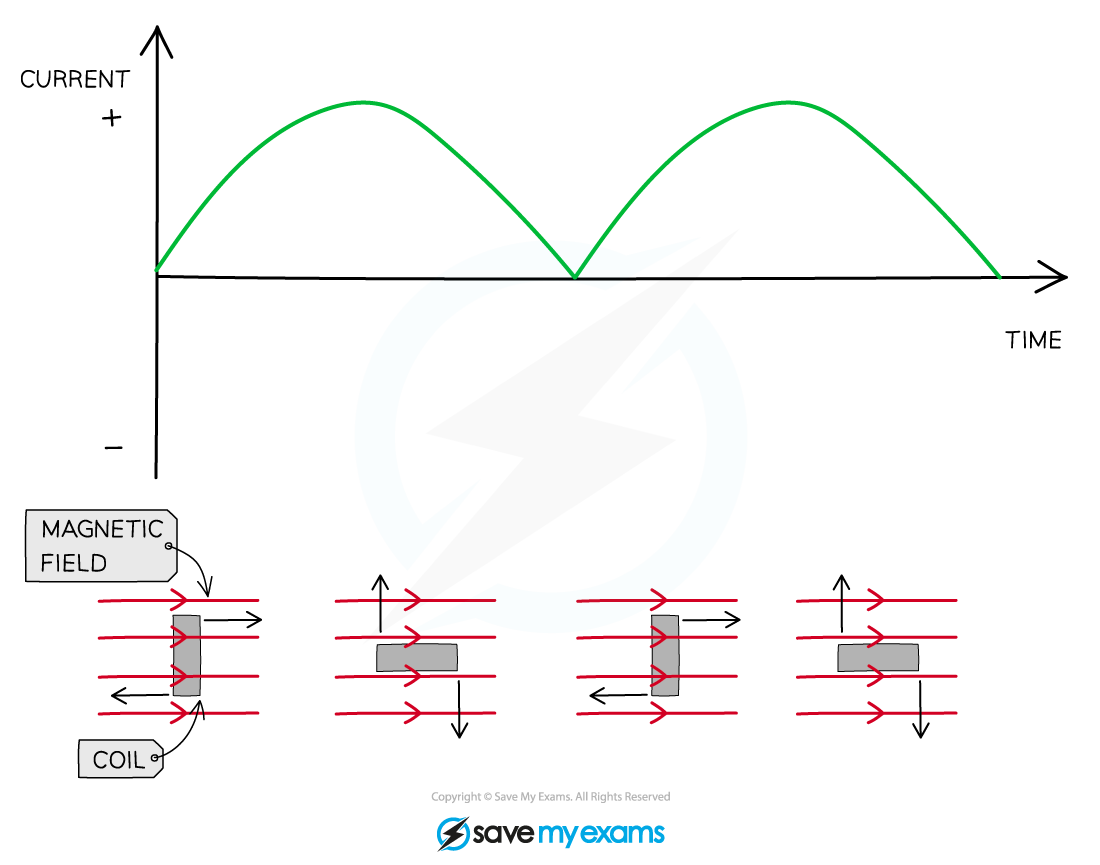# IB DP Physics: HL复习笔记11.2.1 AC Generators

### AC Generators

• If a coil of wire is rotated inside a magnetic field by an external force, an emf will be generated in the wire which causes current to flow within the coil
• The generator effect can be used to:
• Generate a.c. in an alternator
• Generate d.c. in a dynamo

#### Alternators

• A simple alternator is a type of generator that converts mechanical energy to electrical energy in the form of alternating currentAn alternator is a rotating coil in a magnetic field connected to commutator rings

• A rectangular coil that is forced to spin in a uniform magnetic field
• The coil is connected to a centre-reading meter by metal brushes that press on two metal slip rings (or commutator rings)
• The slip rings and brushes provide a continuous connection between the coil and the meter
• When the coil turns in one direction:
• The pointer defects first one way, then the opposite way, and then back again
• This is because the coil cuts through the magnetic field lines and a potential difference, and therefore current, is induced in the coil
• The pointer deflects in both directions because the current in the circuit repeatedly changes direction as the coil spins
• This is because the induced potential difference in the coil repeatedly changes its direction
• This continues on as long as the coil keeps turning in the same direction
• The induced potential difference and the current alternate because they repeatedly change direction

#### Dynamos

• A dynamo is a direct-current generator
• A simple dynamo is the same as an alternator except that the dynamo has a split-ring commutator instead of two separate slip ringsA dynamo is a rotating coil in a magnetic field connected to a split ring commutator

• As the coil rotates, it cuts through the field lines
• This induces a potential difference between the end of the coil
• The split ring commutator changes the connections between the coil and the brushes every half turn in order to keep the current leaving the dynamo in the same direction
• This happens each time the coil is perpendicular to the magnetic field linesD.C output from a dynamo - the current is only in the positive region of the graph

• Therefore, the induced potential difference does not reverse its direction as it does in the alternator
• Instead, it varies from zero to a maximum value twice each cycle of rotation, and never changes polarity (positive to negative)
• This means the current is always positive (or always negative)